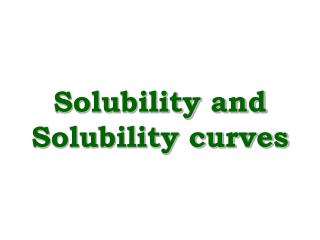DownloadDownload PresentationSolubility and Solubility curves

# Solubility and Solubility curves

Download Presentation## Solubility and Solubility curves

- - - - - - - - - - - - - - - - - - - - - - - - - - - E N D - - - - - - - - - - - - - - - - - - - - - - - - - - -
##### Presentation Transcript

1. Solubility and Solubility curves

2. Solubility • The solubility of a substance is the mass required to make a saturated solution in a given quantity of solvent (usually water) at a certain temperature. • Solubility at a given temperature = maximum mass (g) of a substance that will dissolve in 100 ml of water. • For example at 20°C, the solubility of potassium nitrate is 32 g per 100 g of water.

3. Solubility of a substance is affected by… 1) The nature of the substances For example, ionic compounds and hydrates dissolve in aqueous solutions. 2) The polarity of the substances For example, polar substances dissolve in polar solvents. 3) The temperature Some substances only dissolve above certain temperatures. 4) The pressure Some substances only dissolve when under pressure.

4. Solubility of a solid - KClO3 Draw a graph of the maximum mass of KClO3 dissolved vs. temperature

5. g solute / 100 ml H20

6. Solubility types • Saturated solution – a solution containing the maximum quantity of solute at a specific temperature and pressure • Unsaturated = ? A solution containing less than the maximum quantity of solute possible for that solvent at a specific temp. and pressure. • Supersaturated – ? A solution containing more solute than the saturated conditions.

7. Fig 2 on page 316 Questions 3. To make a saturated solution of KClO3, how much KClO3 is dissolved in 100 ml of water at (i) 20°C (ii) 40°C (iii) 60°C 4. What minimum temperature is needed to dissolve these masses of KNO3 (not KClO3) in 100 ml H2O: (i) 46 g (ii) 15 g (iii) 80 g 5. What type of solution (saturated or unsaturated) forms when ___ g of KClO3 are dissolved in 100 ml of H2O at ___°C: (a) 43 g, 85°C, (b) 32 g, 85°C, (c) 10 g, 60°C. 6. 100 ml H2O is saturated with KClO3 at 100°C. How much KClO3 will come out of solution if the temperature is lowered to 48°C?. How much would precipitate under the same circumstances if 350 ml water were used? 7. A saturated solution of potassium nitrate is cooled from 50°C to 10°C. How many grams of KNO3 will precipitate out of 150 ml of water?

8. Results of lab: solubility of KClO3 g solute / 100 ml H20 KNO3 3a i) 7 Unsaturated ii) 14 Supersaturated 4 i) iii) 24 - 25 Saturated 3b i) 30°C 4 ii) ii) 3°C 4iii) iii) 48°C 4 i) saturated ii) unsaturated iii) unsaturated

9. Saturation and solubility curves See Fig 2 on pg. 316 Notice that there are solubility curves for many different substances Q - Why do temperatures range from 0-100°C A - Water is a liquid at these temperatures Solubility curves tell us how much solid will dissolve at a given temperature They also allow certain calculations…

10. Calculations using solubility curves Using fig 2 (pg. 316) 5. 100 ml H2O is saturatedwith KClO3 at 100°C. How much KClO3 will come out of solution if the temperature is decreased to 48°C? How much would precipitate under the same circumstances if 350 ml water were used 6. 57 g - 18 g = 39 g (57 g - 18 g) x 3.5 = 137 g (85 g - 21 g) x 1.5 = (64 g) x 1.5 = 96 g

11. Solubility of gases • Read page 319 of text ‘Solubility in water – Generalizations and examples’